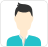true

Find the best tutors and institutes for Class 9 Tuition

Find Best Class 9 Tuition

No matching category found.

No matching Locality found.

Outside India?

# Intext Exercise 11.3

What is the kinetic energy of an object?

Kinetic energy is the energy possessed by a body by the virtue of its motion. Every moving object possesses kinetic energy. A body uses kinetic energy to do work. Kinetic energy of hammer is used in driving a nail into a log of wood, kinetic energy of air is used to run wind mills, etc.Tasneem

Write an expression for the kinetic energy of an object.

If a body of mass m is moving with a velocity v, then its kinetic energyis given by the expression,Its SI unit is Joule (J).Tasneem

The kinetic energy of an object of mass, m moving with a velocity of 5 m s−1 is 25 J. What will be its kinetic energy when its velocity is doubled? What will be its kinetic energy when its velocity is increased three times?

Expression for kinetic energy ism = Mass of the object

v = Velocity of the object = 5 m s−1

Given that kinetic energy,= 25 J

(i) If the velocity of an object is doubled, then v = 5 × 2 = 10 m s−1.

Therefore, its kinetic energy becomes 4 times its original value, because it is proportional to the square of the velocity. Hence, kinetic energy = 25 × 4 = 100 J.

(ii) If velocity is increased three times, then its kinetic energy becomes 9 times its original value, because it is proportional to the square of the velocity. Hence, kinetic energy = 25 × 9 = 225 J.Tasneem

How can we Improve it?

Please enter your question below and we will send it to our tutor communities to answer it *

Name *

Enter a valid name.

Email *

Enter a valid email.

Email or Mobile Number: *